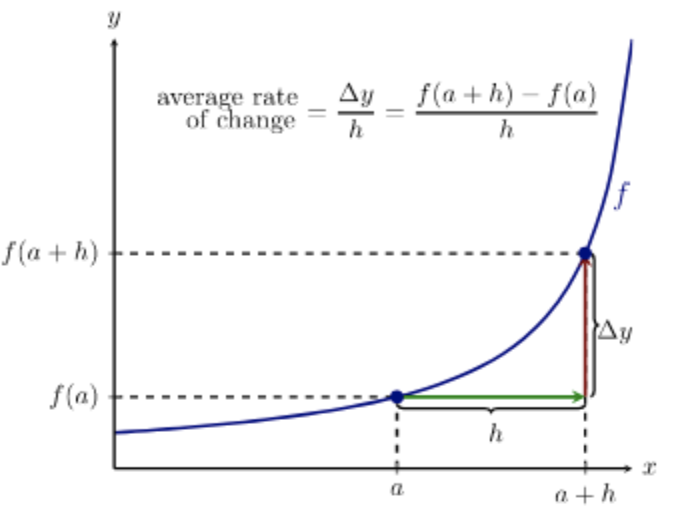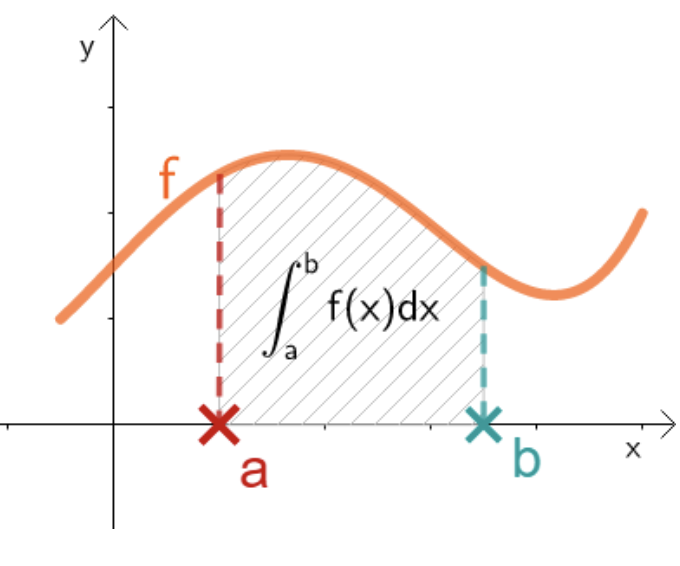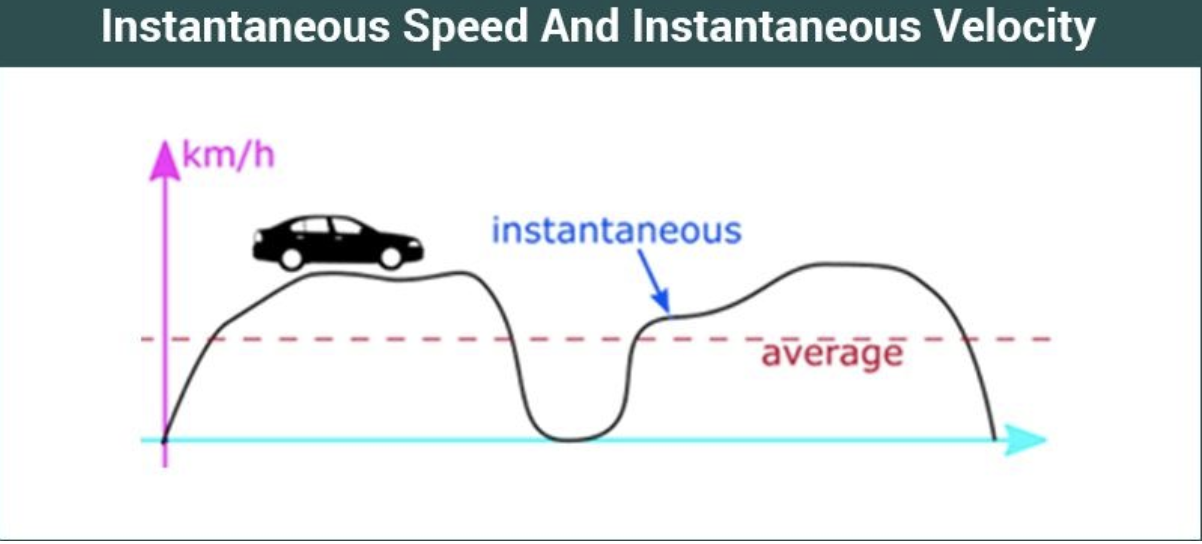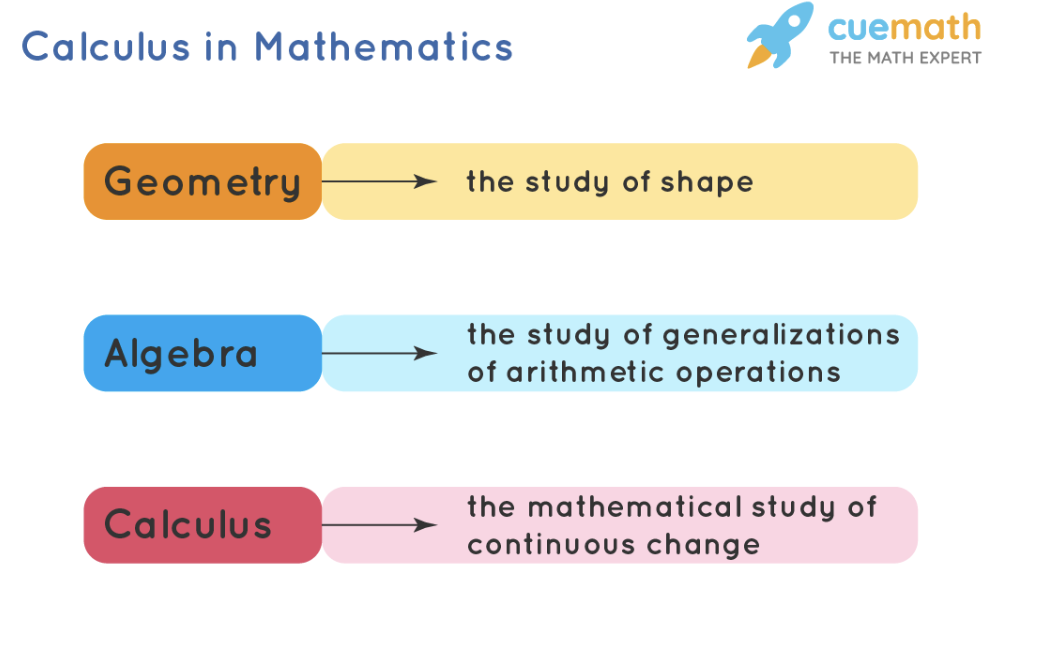# TL;DR Calculus: The key to understanding scienceBy Philip A.
February 10, 2023 · 5 minute read

Mathematics

StatisticsCalculus is widely considered an intimidating subject that you shouldn’t take if you are not a maths person. At first, calculus can appear quite intimidating and abstract. But, this is greatly based on the way it is presented. For most high school students, how you first come in contact with calculus makes or breaks how you perceive it. And this ties in with the latter point about needing to be a ‘maths person’. In this article, I aim to give a short introduction to calculus specifically, why it is important to physics(and generally to all scientific disciplines), and how it started. Lastly, I will try to demystify the two core concepts that you need to understand it.

### Why is calculus important to physics and the sciences?

It would be an understatement to say that calculus is key to understanding the sciences. First of all, calculus lets us model change(e.g. Change of position, change of state, change of direction etc.) in a standardised way which can be taught and applied in any circumstance. That is to say that, if one scientist models a system under specific conditions and mathematically, through calculus, derives a model for that system then any other scientist can use it and it can be taught in a science class. Secondly, the core of calculus is intuitive enough that physical phenomena can be interpreted by any student after having learnt it. Specifically, after having learnt calculus you gain the ability to interpret all sorts of physical phenomena using it, and can successfully model a lot of classical physics phenomena. Finally, while calculus is most important to understanding physics, it applies to all sciences. Whenever you hear the phrases ‘the rate by which something changes’ or ‘how quickly something will happen’ or ‘how can we optimise a process’ you can be sure that calculus is implied in some way.

Some notable examples of where you can find applications of calculus just to name a few are the motion of bodies, oscillations, chemical reaction rates and blood-flow mechanics.

### A brief history of calculus

Calculus was first written down in a systematic way by Sir Issac Newton. There are many examples of how areas of calculus were invented way before Newton but none of them were as complete and as similar to modern-day calculus. The reason that prompted Newton’s discovery was his research into the motion of celestial bodies and his understanding of how gravity works. To do this he worked with a mathematical quantity that he called a fluxion which was basically an infinitesimally small change in a quantity(e.g. position, speed). With this tool and some basic geometry, he was able to interpret and express mathematically how gravity works and how objects move.### The Core Concepts in Calculus

The two core calculus concepts are the derivative and the integral. The shortest explanation that I can give you about what these two concepts are is that a derivative is a slope(in physics speed is a derivative of position) and that an integral is an area under a curve(in physics the integral of speed is position).

Specifically, the derivative is a way of expressing the rate of change of a quantity relative to another. So, if two quantities are related when you change one quantity the other changes as well. But by how much? That is where the derivative comes into play. Let’s take a practical example. An object moving in a straight line has its position changing as time goes by. So at t = 1, it is at x = 1 and at t = 2 it is at x = 3. Because time is continuous, you can infinitely subdivide a second into 0.1 seconds 0.01 seconds and so on. Therefore, there can’t only be one value of how big that change is, but several values one for each specific value of t in this instance. So, in summary, the derivative is a measure of how much the related quantity changes for each value of the parameter quantity. In physics, this is called instantaneous speed and in chemistry, you have instantaneous reaction speeds. This instantaneous rate of change is very useful because it lets the scientist know how much the quantities change per instant of time.On the other hand, the integral assigns a number to a curve that denotes the area over an interval. This area indicates the total change over a period of time. In this, it is different from differentiation as the derivative concerns a single moment in time whereas an integral is concerned with a period of time.Obviously, this is a crude attempt to try and demystify the main concepts. The key takeaway is that both the derivative and the integral express different measures of change. The derivative -to make a physics analogy- is used to calculate the speed at every instant in time whilst the integral is used to calculate the displacement over a period of time.If you don’t want to commit to a full-time calculus course but still want to learn calculus to more thoroughly understand other sciences there is still hope as there is a flurry of videos explaining the intuition that comes along with calculus without the actual computation. I have posted several videos below.### TL;DR

Calculus is a topic that scares many high-school students away and that dissuades many people from learning it. Yet, it plays such an essential role in all sciences especially the physical sciences in that it is a tool to describe change. The two main concepts of calculus are intuitive. They are the derivative and the integral. The former is used to calculate the instantaneous rate of change, in other words, the degree of change at each point and the latter is used to calculate total change over an interval, in other words, the total amount that a quantity has fluctuated.

##### Sources:

Thomas' Calculus 13th Edition